NSWC Crane Home Who We Are What We Do Partnerships Resources Career Opportunities Visitor Information Contact Us EventsIntroduction

The purpose of this document is to familiarize the user with the basic concepts of a de-rating analysis, starting with circuit analysis and proceeding through documentation. Electrical stress de-rating analysis is the process of determining a part's ability to withstand induced stresses under given environmental conditions. Induced stresses are taken from the datasheet and circuit analysis, and are identified in terms of voltage, current, power, etc. Environmental conditions refer primarily to temperature and are taken from the system's operating requirements.

One of the objectives of a stress analysis is to provide early warning of design deficiencies at the time in the program phase where changes are least expensive (i.e., while the design is still on paper). A stress analysis incorporated early into a program can significantly reduce test time and cost by providing a basis for a reliable design.

Transient conditions must also be taken into account. The analysis will not necessarily consider worst case conditions with regard to applied voltages or currents, part parameter values, or driving signals. However, when an undesirable stress condition is noted, worst case conditions should be examined and the probability of worst case occurrence investigated.

Preliminary Analysis

Prior to performing a stress de-rating analysis, a thermal analysis and circuit analysis must first be completed. Circuit analysis is considered outside the scope of this document. In brief, circuit analysis is the process used to calculate the electrical parameters of each part in the circuit, such as voltage, current, power, etc.

Determining Part Stress Ratings

After thermal and electrical parameters are known, it is possible to calculate part stress ratings. The part stress rating is defined as the ratio of applied to rated electrical parameters. These electrical parameters may consist of voltage, current, power, inverse voltage, etc., or any combination, depending on the part. For example, a power stress ratio is used for a resistor, while a voltage stress ratio is used for a capacitor.

A part's stress rating can vary from lot to lot, vendor to vendor, application, and nearly always increases with increasing temperature. This document will concentrate on the latter; that is, how to calculate stress ratings with increasing temperatures. It will do this by presenting examples. For the purpose of these examples, it is assumed a thermal profile analysis has already been done in accordance with the Thermal Analysis document.

Examples

Example #1: Application of a Solid Tantalum Capacitor Voltage Rating

Given:

a.  The device is a 6.8 mf electrolytic tantalum capacitor. Device ratings according to the datasheet are shown in Figure 1. Maximum rated operating voltage is 25 VDC up to 85oC. Absolute maximum operating temperature (TMAX) is 125oC. The device is rated for operation up to 16 VDC of maximum operating at 125oC.

b. Maximum equipment external ambient temperature profile is 70oC. The internal temperature rise in the equipment was determined to be 30oC using the principles of the Thermal Analysis document.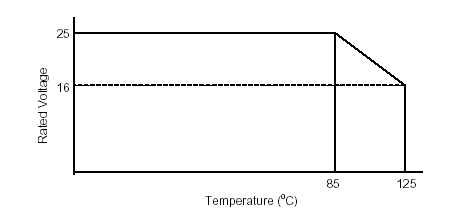Figure 1: Maximum Voltage Rating for Electrolytic Tantalum Capacitor, Example #1

c.  Maximum impressed voltage is 10 VDC, determined from the electrical circuit analysis.

Determine:

a.  The maximum voltage rating of the capacitor at the operating temperture.

b.  The required capacitor voltage rating using the derating requirements in this manual.

Solution:

First, determine the part ambient operating temperature (Top). This is the sum of the external ambient equipment temperature (70oC) and the internal temperature rise (30oC) or,

Top = 70oC + 30oC = 100oC

At an operating temperature of 100oC, the maximum part rating is determined by one of formulas, depending on the operating temperature (Top):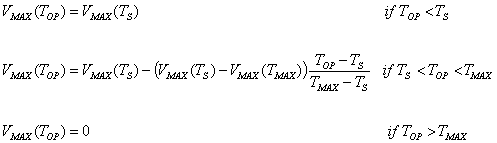Eq-1

The operating temperature (Top) given in the example (100oC) is between Ts (85oC) and TMAX (125oC), so the center equation is used. Substituting: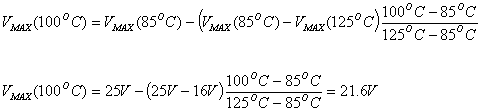O some users prefer to use the slope version of the formula instead, as follows, which yields identical results: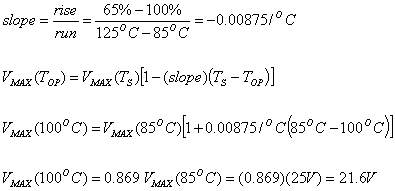Eq-2

For the solution to part (b) of the question, first determine the derating guidelines for tantalum capacitors. The derating guidelines for electrolytic tantalum capacitors 60% to Ts, and then linearly derated to TMAX - 10oC. This is reproduced in Figure 2. Also shown is the operating temperature of the capacitor along with its maximum allowed stress ratio.

The maximum derated Stress Ratio (SRD) at the operating temperature is calculated by the formula: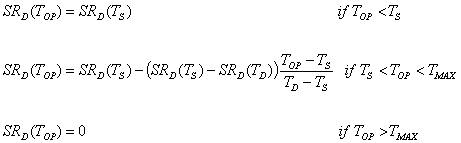Eq-3

(Note this is identical to Eq-1 on the previous page except stress ratio is substituted for voltage.)

Substituting: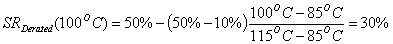The current 25V capacitor therefore does not meet the derating guidelines. The derated maximum rating is greater than the actual voltage the capacitor is expected to see, or: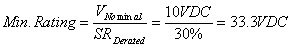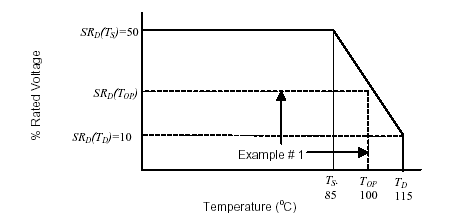Figure 2: Derating Requirements For Electrolytic Tantalum Capacitor,
Example #1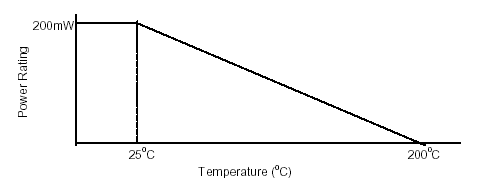Figure 3: Maximum Power and Temperature Ratings Transistor,
Example #2

To meet the derating guidelines, a capacitor with a voltage rating of at least 33.3 VDC is required, calculated as follows:The proper capacitor is 6.8 mf, 35 VDC rated.

Example #2, Application of a Transistor

Given:

A user requires a transistor to be used in an application where it will be required dissipate 70 mW of power. Ambient temperature (TA) in the immediate vicinity of the transistor is 50oC.

The selected transistor has maximum power dissipation ratings shown in Figure 3. The transistor can dissipate up to 200mW at 25oC. Above 25oC, the datasheet requires the maximum power dissipation rating to be linearly "derated" to a maximum junction temperature (TJ) of 200oC. The transistor has a thermal resistance (QJA) from junction to ambient of 0.875oC/mW.

Determine:

Determine if the selected resistor can be properly derated for the given application using the derating guidelines of this document.

Solution:

The derating guidelines for a silicon transistor are reproduced in Figure 4. Ts and TMAX are taken from the datasheet and the given information. Ts is the maximum operating temperature at which full rated power can be applied, or in this case 25oC. TMAX is the maximum junction temperature (TJ) or absolute maximum temperature at which the part can operate. In this case, it is 200oC. Maximum derated temperature (TD) is then calculated by the formula: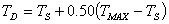Substituting,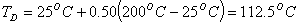Eq-4

The derating curve is now completely defined and shown in Figure 4.

Next, determine the derated stress ratio (SRD) allowed by the derating guidelines. Equation #3 is again used, except it is simplified because the derated stress ratio is zero at TMAX, or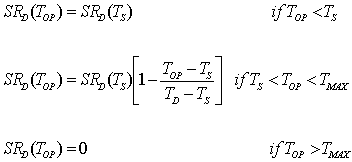Eq-5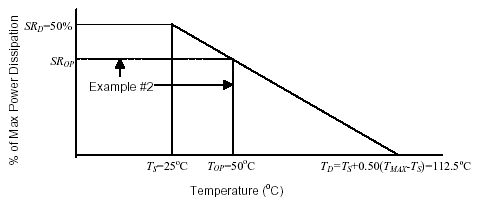Figure 4: Transistor Derating Requirements, Example #2.

Operating temperature is 50oC. This is between Top (25oC) and Ts (125oC), so the center equation is used. Substituting,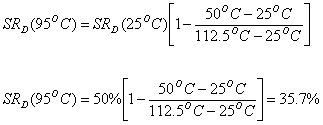The stress ratio allowed by the derating guidelines at an ambient temperature of 50oC is therefore 35.7% of the maximum power rating. Since the maximum power rating was given at 200 mW, the transistor is not allowed in an application where it dissipates more than 71.4 mW. Since its actual power dissipation was given at 70 mW, the transistor meets the derating guidelines.

Alternative Solution:

An alternate method of determining if the transistor exceeds its derating guidelines is to determine if actual junction temperature exceeds derated junction temperature.

Actual junction temperature is calculated using the thermal resistance properties of the device. The datasheet gave the thermal resistance, junction to ambient (QJA), as 0.875oC/mW. We also know the transistor is dissipating 70mW of power. Junction temperature can therefore be calculated using the equation: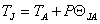Substituting: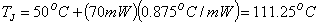The derated junction temperature was previously calculated in Eq-4 to be 112.5oC. Since actual junction temperature does not exceed maximum derated junction temperature, the transistor is acceptable to use in the given application.

Note: the reason this short cut can be used is because the slope of the derating lines in both Figure 3 and Figure 4 is equal to the inverse of the thermal resistance. In other words, the junction temperature at any point along the derating line will be the same.

Documenting The Stress Analysis

The next step in the electrical stress derating analysis process is to calculate the stress ratios and document the results of the stress analysis. The stress ratio is the numeric ratio between the actual stresses determined from the circuit analysis divided by the stress rating of the part at the operating temperature. This can be expressed mathematically as a percentage as follows:

The best method to document a stress analysis is usually through a worksheet or spreadsheet that allows for a logical flow of information from left to right with all required data and parameters specifically called out in columns. This obviates the casual omission of required parameters. the analysis is most effectively accomplished with the use of different worksheets for the various part categories. Many facilities perform the stress analysis, derating analysis, and reliability prediction all in a single spreadsheet to increase efficiency. It is also possible to generate macros to perform much of the repetitive work. Examples of typical worksheets are contained in the following pages containing Tables 1 through 6.

 Equipment Schematic                         No.                      Rev Title: Max Alt: (ft) Next Assy                        No. Title: Ref Des (type) Const- Struction Type Vendor Procur Doc Nom Resist Mfg Tol TA Voltage Power Dissipation Percent Power Rated Percent Voltage Rated Wave Form (dc, sine, pulse, etc) Remarks Resistive Element Nom Rated Actual Rated 25oC Rated Max Amp Actual W % oC V V mW mW mW % V Report Number Rev                Date Proj Appd          Date Appd             Date Project               No. Sheet          ____ of ____

Table 1: Part Usage and Applied Stress Data Chart for Resistors.

 Equipment Schematic                         No.                      Rev Title: Max Alt: (ft) Next Assy                        No. Title: Ref Des # Const Dielec- tric Cap Type Vendor Procur Doc Part Amb Temp Cap Value Mfg Tol Voltage % Volt Rating Wave Form (dc, sine, pulse, etc) Remarks Rated Operating 25oC TD DC Peak AC RMS Freq Pulse Peak Rep Rate oC nF % V V VDC V V KHz V ms % Report Number Rev                Date Proj Appd      Date Appd          Date Project        No. Sheet          ____ of ____

Table 2: Part Usage and Applied Stress Data Chart for Capacitors.

 Equipment Schematic                                            Title: No.               Rev Max Alt: (ft) Next Assy                         Title: No. Ref Des # Vendor Part # Vend Procur Doc Type GP, Power, etc. Part Amb Temp Contact Arrage- ment (form) Contact Loading Coil Voltage % Cont Power Rating % Cont Curr Rating % Coil Max Volt Rating % Coil Pick Volt % Coil Drop Volt Con- tact Power Current Load Maximum Pickup Dropout Nom Rated Act Nom Rated Act Rated Act res/ind cap Nom Rated Act Rated Act Nom Rated Act oC oC mW mW mA mA mV mV mV mV mV mV % % % % % Report Number Rev                            Date Proj Appd              Date Appd                  Date Project                  No. Sheet               ____ of ____

Table 3: Part Usage and Applied Stress Data Chart for Relays.

 Equipment Schematic                         No.                      Rev Title: Max Alt: (ft) Next Assy                        No. Title: Ref Des # Vendor Part # Vendor Procur Doc Type Si Ge Max Amb Temp Voltage Peak Inverse Voltage Forward Current Percent Current Rating Percent PIV Rating Q TJ Notes Rated 25oC Rated Max Ambient Actual Max Rated Actual Max Rated Actual oC V V V V V mA mA % % oC/W oC Report Number Rev                    Date Proj Appd           Date Appd                  Date Project                No. Sheet                  ____ of ____

Table 4: Part Usage and Applied Stress Data Chart for Zener Diodes.

 Equipment Schematic                         No.                      Rev Title: Max Alt: (ft) Next Assy                        No. Title: Ref Des (type) Vendor  Part # Vendor Procur Doc Type Si Ge Max Amb Temp Power Dissipation Peak Inverse Voltage Forward Current Percent Current Rating Percent PIV Rated Remarks Rated 24oC Rated Max Ambient Actual Max Rated Actual Max Rated Actual oC mW mW mW V V A A % % Report Number Rev                   Date Proj Appd               Date Appd             Date Project                  No. Sheet          ____ of ____

Table 5: Part Usage and Applied Stress Chart for General Purpose and Power Rectifier Diodes.

 Equipment Schematic                                       Title: No.                         Rev Max Alt: (ft) Next Assy                         Title: No. Ref Des # Vendor Part # Vend Proc Doc Part Amb Temp Power Dissipation Voltage Current Perc Output Rating Perc Supply Rating Perc Current Rating Perc lc Rating Q Res TJ Rated Actual Input Output Supply Collector Perc Input Rating @ 25oC @ TD Rated Act Rated Act Rated Act Rated Act oC mW mW mW V V V V V V mA mA % % % % % oC/W (oC) Report Number Rev              Date Proj Appd              Date Appd                  Date Project                         No. Sheet               ____ of ____

Table 6: Part Usage and Applied Stress Data Chart for Linear Microcircuits